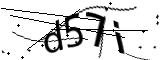Ordering Information

Figuring Concrete

Ready mixed concrete is ordered by the cubic yard.  One cubic yard of concrete equals 27 cubic feet--or the amount of concrete that would fill a container that is 3 feet wide * 3 feet long * 3 feet deep.  To determine the amount of concrete for your specific project, calculate the in place volume, in feet, by first multiplying the lengt by the width, which will give you the total square feet.  Then take that number and multiply it by the depth or thickness of your pour, also in feet; keeping in mind that 4 inches is equal to .333 feet.  Next, divide that number by 27 (the number of cubic feet in a cubic yard) to get cubic yards.

You can also use the following tool to calculate it for you.
Of course, depending on how accurate your measurements are, how uneven the bottom surface of your project area might be, how much space any pipes, columns, or reinforcing material might occupy, and how much might be spilled during placement, actual amounts may vary.

Note: There is a minimum delivery of one yard of concrete.

•Reload